maths > fractions

Division of Fractions

what you'll learn...

overview

Fractions are part of whole. Division is dividing a number into equal parts.

•  division in first principles -- splitting a quantity into a number of parts and measuring one part

•  simplified procedure : Division as multiplication by reciprocal

just know this

Division is the inverse of multiplication. And division of fractions can be easily explained with that.

$\frac{3}{4}÷\frac{2}{3}$$\frac{3}{4} \div \frac{2}{3}$
$\quad = \frac{3}{4} \times \frac{3}{2}$ (multiply by inverse)
$\quad = \frac{9}{8}$.

A learner may stop at this, but I would suggest to read the rest (too long, too hard) to understand how division works for fractions.

or understand this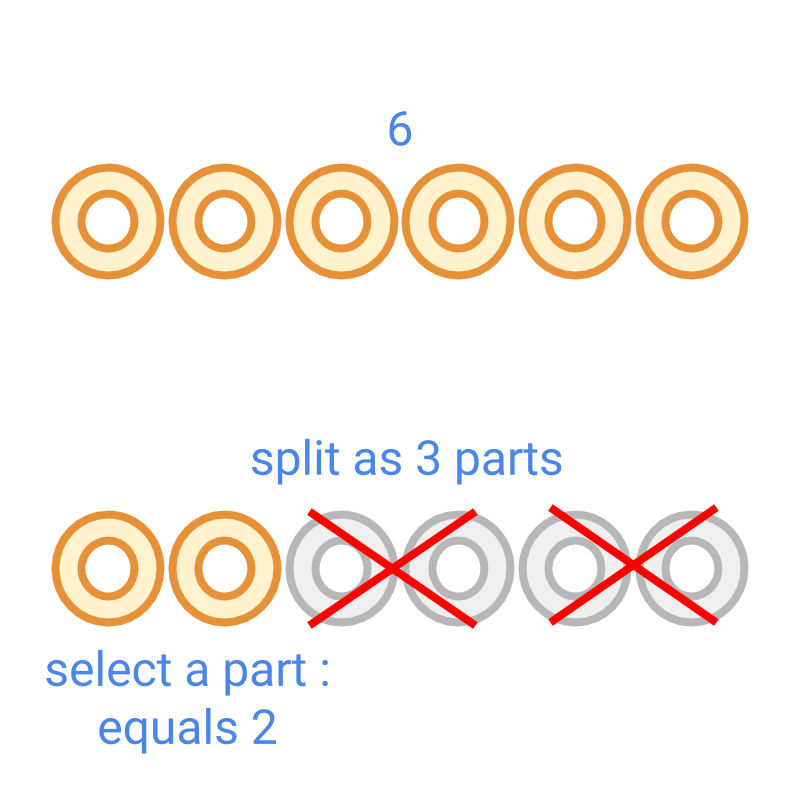Division $6÷3=2$$6 \div 3 = 2$ is illustrated in the figure.

• $6$$6$ is the dividend

• $3$$3$ is the divisor

• $2$$2$ is the quotient

Dividend $6$$6$ is split into divisor $3$$3$ parts and one part of that $2$$2$ is the quotient.

One way to understand the integer division:

•  Dividend $6$$6$ is considered as $3$$3$ parts

•  in that $3$$3$ is the divisor

•  In the $3$$3$ parts one part is taken.

The key in this explanation is " dividend is considered as divisor parts and one part is taken". The same can be extended for fractions.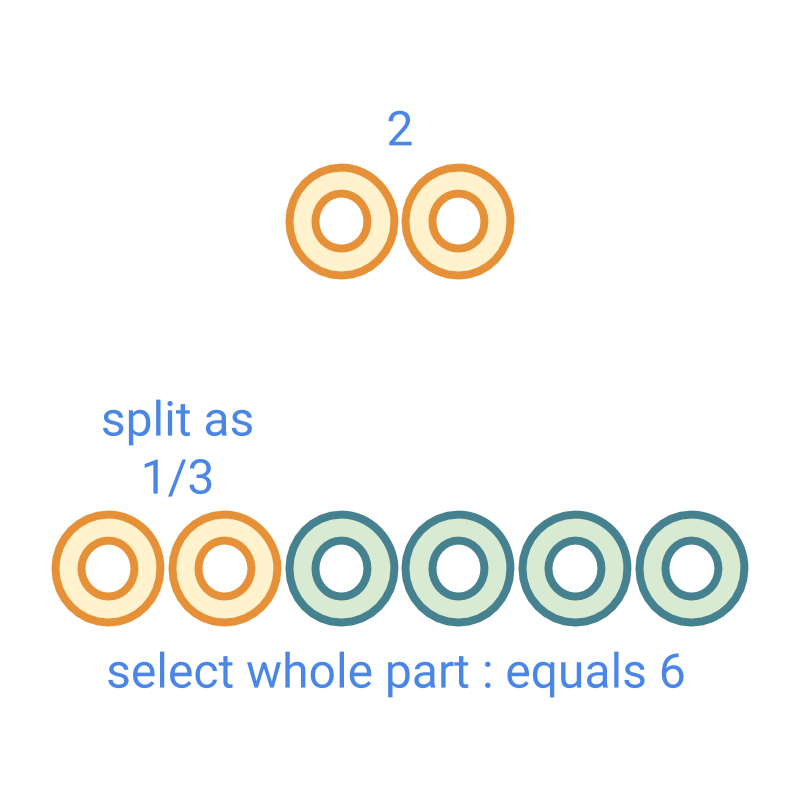Division $2÷\frac{1}{3}$$2 \div \frac{1}{3}$ is illustrated in the figure.

Dividend $2$$2$ is split as divisor $\frac{1}{3}$$\frac{1}{3}$ parts. That is $2$$2$ is the fraction $\frac{1}{3}$$\frac{1}{3}$ and the full part for the fraction is found. The $\frac{1}{3}$$\frac{1}{3}$ part is repeated to get the full part. This is shown in the figure.

In this, one part $6$$6$ is the quotient. $2÷\frac{1}{3}=6$$2 \div \frac{1}{3} = 6$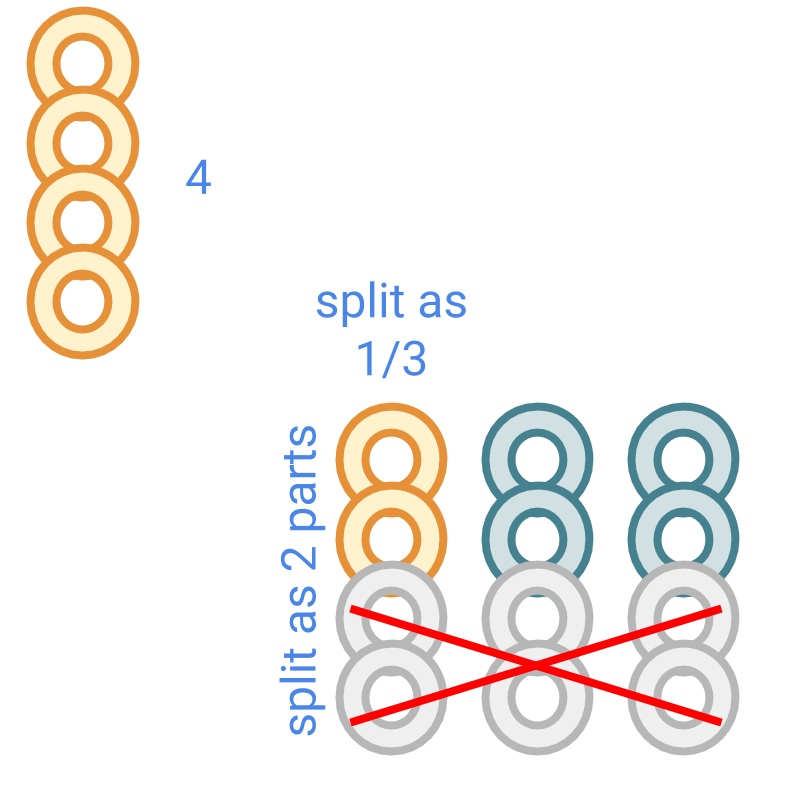Division $4÷\frac{2}{3}$$4 \div \frac{2}{3}$ is illustrated in the figure.

Dividend $4$$4$ is split as divisor $\frac{2}{3}$$\frac{2}{3}$ parts.

In this, one part $6$$6$ is the quotient.
$4÷\frac{2}{3}=6$$4 \div \frac{2}{3} = 6$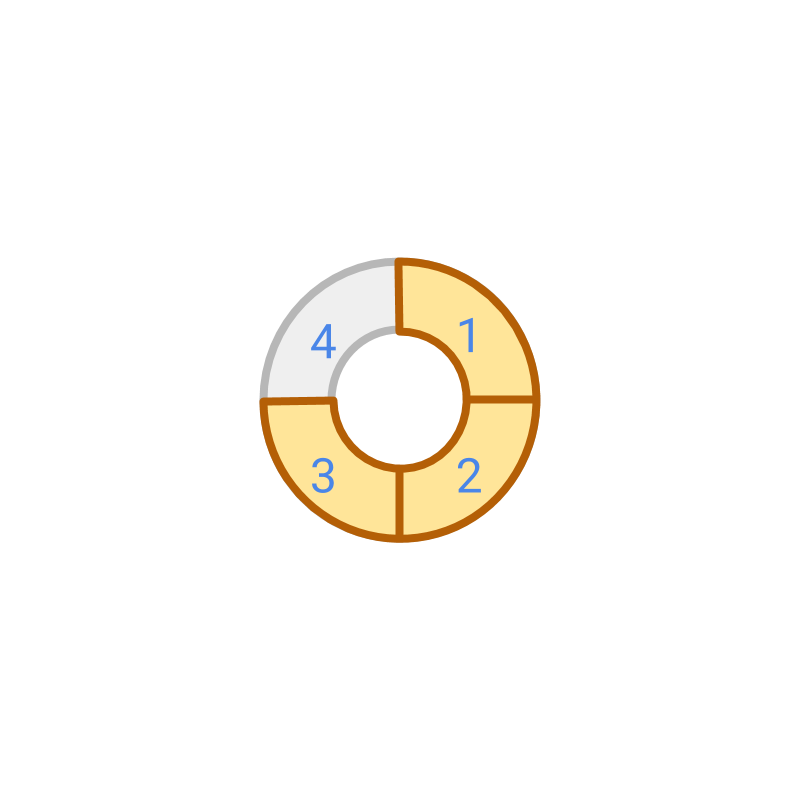Division $\frac{3}{4}÷\frac{2}{3}$$\frac{3}{4} \div \frac{2}{3}$ is considered. The figure shows the dividend $\frac{3}{4}$$\frac{3}{4}$. The division is illustrated in the next page.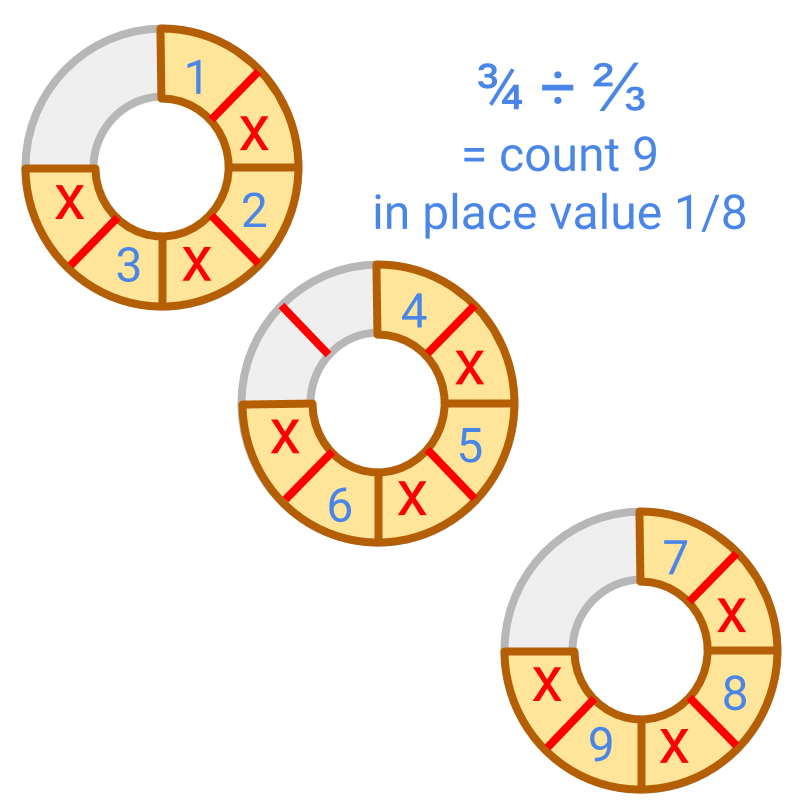Division $\frac{3}{4}÷\frac{2}{3}$$\frac{3}{4} \div \frac{2}{3}$ is illustrated in the figure.

Dividend $\frac{3}{4}$$\frac{3}{4}$ is split as divisor $\frac{2}{3}$$\frac{2}{3}$ parts. This is shown in the figure.

In this, the place value is $\frac{1}{8}$$\frac{1}{8}$ and the count is 9.
The same can be simplified as follows.

$\frac{3}{4}÷\frac{2}{3}=\frac{3}{4}×\frac{3}{2}=\frac{9}{8}$$\frac{3}{4} \div \frac{2}{3} = \frac{3}{4} \times \frac{3}{2} = \frac{9}{8}$

yeah! enough of that

Division is inverse of multiplication.

Division of Fractions: Given two fractions $\frac{p}{q}$$\frac{p}{q}$ and $\frac{l}{m}$$\frac{l}{m}$, the division is
$\frac{p}{q}÷\frac{l}{m}$$\frac{p}{q} \div \frac{l}{m}$
$\quad = \frac{p}{q} \times \frac{m}{l}$
$\quad = \frac{p \times m}{q \times l}$

example

Divide $\frac{14}{18}÷\frac{4}{3}$$\frac{14}{18} \div \frac{4}{3}$.
The answer is '$\frac{7}{12}$$\frac{7}{12}$'

summary

»  $\frac{a}{b}÷\frac{c}{d}$$\frac{a}{b} \div \frac{c}{d}$ = $\frac{a}{b}×\frac{d}{c}$$\frac{a}{b} \times \frac{d}{c}$

»  $6÷3$$6 \div 3$→  $6$$6$ is $3$$3$ parts and find the group representing $1$$1$ part
→  $=2$$= 2$

»  $2÷\frac{1}{3}$$2 \div \frac{1}{3}$→  $2$$2$ is $\frac{1}{3}$$\frac{1}{3}$ part and find the group representing $1$$1$ part
→  $=6$$= 6$

»  $\frac{3}{4}÷\frac{2}{3}$$\frac{3}{4} \div \frac{2}{3}$→  $\frac{3}{4}$$\frac{3}{4}$ is $\frac{2}{3}$$\frac{2}{3}$ part and find the group representing $1$$1$ part
→  $=\frac{9}{8}$$= \frac{9}{8}$

Making sense of the first principles of division by fraction is one of the exhilarating experiences of learning.

»  Procedural Simplification: Division is multiplication by inverse
→  $\frac{3}{4}÷\frac{2}{3}$$\frac{3}{4} \div \frac{2}{3}$
→  $=\frac{3}{4}×\frac{3}{2}$$= \frac{3}{4} \times \frac{3}{2}$
→  $=\frac{9}{8}$$= \frac{9}{8}$

Outline